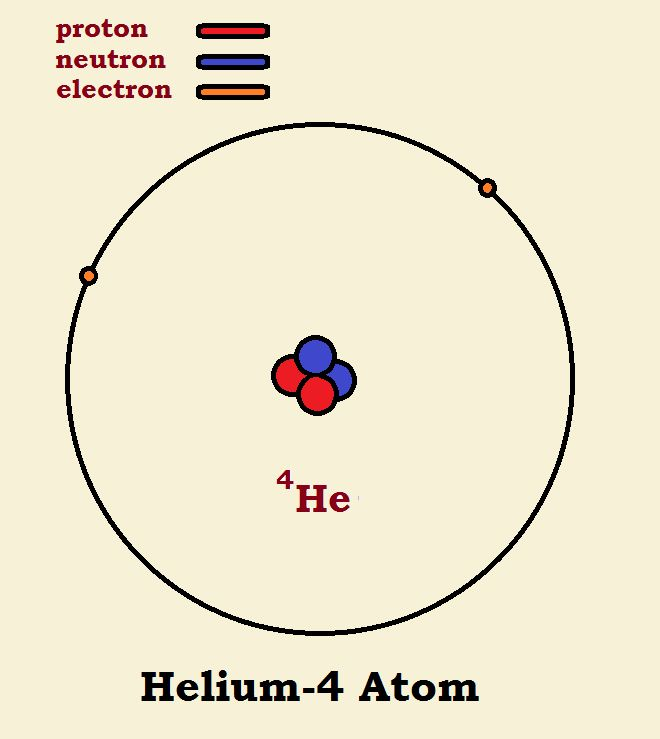# Comparing ⁴He with ²H₂ – An Atom with a Molecule?The title of this article may seem a bit strange. How do we begin comparing a helium-4 atom with a molecule of deuterium hydrogen? It may become more apparent if we examine illustrations of the two species. Note the similarities and the differences. If you do, comparing 4He with 2H₂ should not seem so strange.

## Helium-4

Helium is element number two in the periodic table. This means helium has an atomic number of two—it has two protons. Protons are positively charged. Now an atom is neutral, thus electrical neutrality dictates a helium atom also has two electrons. The only variable is the number of neutrons. Helium-4 (sometimes written 4He) is a very stable form of helium and has two neutrons.

## Deuterium or Heavy Hydrogen

Atomic hydrogen (symbol H) consists primarily of atoms with a central nucleus consisting of a single proton, encompassed by a spherical orbital containing one electron. But some hydrogen atoms are different, as for instance the so-called heavy hydrogen, or deuterium (symbol D). The proton in this variety of hydrogen is joined by a neutron, which approximately doubles the weight.## Hydrogen Molecules

When two hydrogen atoms form a molecule, the two nuclei remain intact, but the electrons are located in a single orbital. This orbital is not spherical, but elliptical. Within the orbital ellipse, the two nuclei are located at the focal points. The major isotope of hydrogen molecules has no neutrons in the nucleus. Its formula is written H₂ or 1H₂. The 1 stands for one proton in the nucleus (hence, one nucleon). Deuterium hydrogen has one neutron in its nucleus. Its formula is written D₂ or 2H₂. The 2 stands for two nucleons—one proton plus one neutron.

## Comparing 4He with 2H₂

Carefully note the differences shown in the diagram. Both 4He and 2H₂ have 2 protons, 2 neutrons, and 2 electrons. One has one nucleus or focal point at the center of the sphere. The other has two nuclei at the focal points of the ellipse. The difference in this is that in the deuterium the nuclei have not been fused into one.

## Energy Discussion

A discussion of energy should prove most interesting. Why? Because the total energy of a system is often generally unavailable. We will compare

1. The chemical energy available from the hydrogen and helium.
2. The available nuclear energy by conversion of one into the other.
3. The total energy available if the helium and the hydrogen are annihilated.

## Chemical Energy

We’ll make quick work of this one. Helium is essentially non-reactive. Deuterium, on the other hand, is moderately reactive. For instance, deuterium reacts with chlorine gas to form the chloride. It is, of course, chemically nearly identical to the formation of ordinary hydrogen chloride from hydrogen gas and chlorine gas.

## Nuclear Energy

We can also make moderately quick work of this one. It is well known that large atoms, split apart, produce smaller atoms with the release of energy, sometimes awe-inspiring energy, as is the case for the uranium bomb. As the size of the atom to be split decreases, however, the theoretical energy released decreases, until one reaches a kind of null point upon reaching the element iron.

Conversely, if tiny molecules are fused, energy is released, yes, even awe-inspiring energy, as is the case with both the hydrogen bomb and its natural counterpart, the stars such as our Sun. As one approaches iron, once again, a null point is reached where energy release fades away. So can we give a theoretical derivation that demonstrates a molecule of deuterium would release energy if it could be fused? Consider the total energy calculations, below.## Total Energy

The total energy of an atom or molecule can be calculated from its mass. Now both the helium and the deuterium in our discussion have the same variety and number of constituent subatomic particles, that is protons, neutron, and electrons. Their energy varies a little. We determine how much using Einstein’s equation, E = mc², where E is the energy, m is the mass, and c is the speed of light.

The atomic mass for 4He is 4.0026 unified atomic mass units, or 4.0026u. A molecule of deuterium is 4.0282 unified atomic mass units. We convert to grams by multiplying by 1.660539 x 10-24. We provide the calculations in a diagram. Note especially, the conclusion in the box at the bottom of the diagram.

## Closing Remarks

In conclusion, in comparing 4He with 2H₂ we suggested the chemical stability of helium might be of lower energy than its counterpart, deuterium. The calculation of total energy reveals we were correct in our assumption, and those calculations also give solid evidence converting deuterium to helium-4 via fusion would release considerable energy.

Note: You might also enjoy Hydrogen Metal?

References:

### 2 thoughts on “Comparing ⁴He with ²H₂ – An Atom with a Molecule?”

• That was really interesting and very clearly explained (although I did not check out the maths, LOL). Is this the type of fusion that scientists have been trying to produce in the lab?

• They’re interested in so-called cold fusion. The same basic idea with a twist…